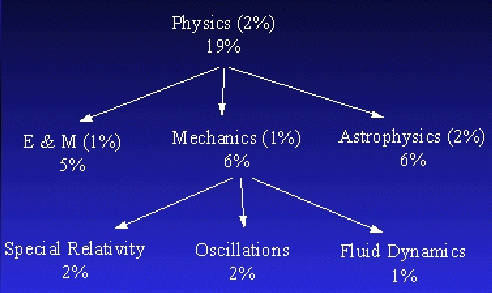### Appendix C -- Automatically Classifying the Newsgroups

Once we have constructed the LSI term vector space, we use this data to characterize newsgroups within the LCC. Each newsgroup, which is treated as a separate collection, requires its own profile. A profile is compiled by processing the individual news articles within each newsgroup. We first pre-process each article, mainly to strip its headers (and remove punctuation). The only header information we keep is the content of the subject line, which in principle the author wrote intentionally to describe the message's content.LSI queries take the form of new documents or free text. The terms of the query define a new vector in the LCC vector space in the same manner as the original documents. LSI then returns a weighted list of similar documents. In our case, this list is a set of LCC categories whose weights indicate some measure of relevance between the query terms and the categories. In effect, this procedure automatically classifies the query into the LCC. As shown in Figure 3, each news article is given as a query to LSI, which returns the weighted list of LCC nodes.We then keep all nodes which have a weight above a threshold value (currently 0.25). Suppose we are returned four nodes with the following weights: Node A: 0.8; Node B: 0.8; Node C: 0.4; and Node D: 0.1. We first drop Node D, since its weight is below our threshold value. We then normalize the remaining weights so that exactly 100% of the article is divided between the remaining nodes; thus the article is assigned 40% to Node A, 40% to Node B, and 20% to Node C.For each newsgroup, we construct the classification-based collection profile required by Pharos. In this case, this profile takes the form of an LCC tree where each node contains the percentage of articles in that newsgroup associated with that node in the tree. As an example, Figure 4 shows a collection profile where 19% of the collection falls under the Physics node or its children in the classification, 6% falls under Mechanics, etc. The values in parentheses denote articles which fall under a particular node but not under any of that node's children.

We process each article in the newsgroup as above, and then allocate it among the nodes such that 100% of it is added in. If we assume that there are 10 documents in a collection, then each document adds a total of 0.1 to its collection profile. Hence, if we were adding the article from the above example to this profile, we would add 0.04 to the document count of Node A, 0.04 to Node B, and 0.02 to Node C. After processing each article in a newsgroup, we end up with the total number of document equivalents associated with each of the 4214 nodes in the LCC tree. One newsgroup profile is generated in this manner for each newsgroup.